# 12 java.lang.Math Functions

This chapter provides a reference to the `java.lang.Math` functions provided in Oracle Continuous Query Language (Oracle CQL).

This chapter includes the following section:

## 12.1 Introduction to Oracle CQL Built-In java.lang.Math Functions

Table 12-1 lists the built-in `java.lang.Math` functions that Oracle CQL provides.

Table 12-1 Oracle CQL Built-in java.lang.Math Functions

Type Function

Trigonometric

• `sin`

• `cos`

• `tan`

• `asin`

• `acos`

• `atan`

• `atan2`

• `cosh`

• `sinh`

• `tanh`

Logarithmic

• `log1`

• `log101`

• `log1p`

Euler's Number

• `exp`

• `expm1`

Roots

• `cbrt`

• `sqrt`

• `hypot`

Signum Function

• `signum`

• `signum1`

Unit of Least Precision

• `ulp`

• `ulp1`

Other

• `abs`

• `abs1`

• `abs2`

• `abs3`

• `ceil1`

• `floor1`

• `IEEEremainder`

• `pow`

• `rint`

• `round`

• `round1`

• `todegrees`

• `toradians`

Note:

Built-in function names are case sensitive and you must use them in the case shown (in lower case).

Note:

In stream input examples, lines beginning with `h` (such as `h 3800`) are heartbeat input tuples. These inform Oracle Event Processing that no further input will have a timestamp lesser than the heartbeat value.

## abs

Syntax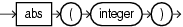Purpose

`abs` returns the absolute value of the input `integer` argument as an `integer`.

For more information, see `http://java.sun.com/javase/6/docs/api/java/lang/Math.html#abs(int)`.

Examples

Consider the query `q66` in Example 12-1. Given the data stream `SFunc` with schema `(c1 integer, c2 double, c3 bigint)` in Example 12-2, the query returns the stream in Example 12-3.

Example 12-1 abs Function Query

```<query id="q66"><![CDATA[
select abs(c1) from SFunc
]]></query>
```

Example 12-2 abs Function Stream Input

```Timestamp   Tuple
10         1,0.5,8
1000        -4,0.7,6
1200        -3,0.89,12
2000         8,0.4,4
```

Example 12-3 abs Function Stream Output

```Timestamp   Tuple Kind  Tuple
10:       +           1
1000:       +           4
1200:       +           3
2000:       +           8
```

## abs1

Syntax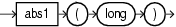Purpose

`abs1` returns the absolute value of the input `long` argument as a `long`.

For more information, see `http://java.sun.com/javase/6/docs/api/java/lang/Math.html#abs(long)`.

Examples

Consider the query `q67` in Example 12-4. Given the data stream `SFunc` with schema `(c1 integer, c2 float, c3 long)` in Example 12-5, the query returns the stream in Example 12-6.

Example 12-4 abs1 Function Query

```<query id="q67"><![CDATA[
select abs1(c3) from SFunc
]]></query>
```

Example 12-5 abs1 Function Stream Input

```Timestamp   Tuple
10        1,0.5,8
1000        4,0.7,-6
1200        3,0.89,-12
2000        8,0.4,4
```

Example 12-6 abs1 Function Stream Output

```Timestamp   Tuple Kind  Tuple
10:       +           8
1000:       +           6
1200:       +           12
2000:       +           4
```

## abs2

Syntax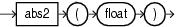Purpose

`abs2` returns the absolute value of the input `float` argument as a `float`.

For more information, see `http://java.sun.com/javase/6/docs/api/java/lang/Math.html#abs(float)`.

Examples

Consider the query `q68` in Example 12-7. Given the data stream `SFunc` with schema `(c1 integer, c2 float, c3 bigint)` in Example 12-8, the query returns the stream in Example 12-9.

Example 12-7 abs2 Function Query

```<query id="q68"><![CDATA[
select abs2(c2) from SFunc
]]></query>
```

Example 12-8 abs2 Function Stream Input

```Timestamp   Tuple
10        1,0.5,8
1000        4,-0.7,6
1200        3,-0.89,12
2000        8,0.4,4
```

Example 12-9 abs2 Function Stream Output

```Timestamp   Tuple Kind  Tuple
10:       +           0.5
1000:       +           0.7
1200:       +           0.89
2000:       +           0.4
```

## abs3

SyntaxPurpose

`abs3` returns the absolute value of the input `double` argument as a `double`.

For more information, see `http://java.sun.com/javase/6/docs/api/java/lang/Math.html#abs(double)`.

Examples

Consider the query `q69` in Example 12-10. Given the data stream `SFunc` with schema `(c1 integer, c2 float, c3 bigint, c4 double)` in Example 12-11, the query returns the stream in Example 12-12.

Example 12-10 abs3 Function Query

```<query id="q69"><![CDATA[
select abs3(c4) from SFunc
]]></query>
```

Example 12-11 abs3 Function Stream Input

```Timestamp   Tuple
10        1,0.5,8,0.25334
1000        4,0.7,6,-4.64322
1200        3,0.89,12,-1.4672272
2000        8,0.4,4,2.66777
```

Example 12-12 abs3 Function Stream Output

```Timestamp   Tuple Kind  Tuple
10:       +           0.25334
1000:       +           4.64322
1200:       +           1.4672272
2000:       +           2.66777
```

## acos

Syntax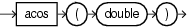Purpose

`acos` returns the arc cosine of a `double` angle, in the range of `0.0` through `pi`, as a `double`.

For more information, see `http://java.sun.com/javase/6/docs/api/java/lang/Math.html#acos(double)`.

Examples

Consider the query `q73` in Example 12-13. Given the data stream `SFunc` with schema `(c1 integer, c2 double, c3 bigint)` in Example 12-14, the query returns the stream in Example 12-15.

Example 12-13 acos Function Query

```<query id="q73"><![CDATA[
select acos(c2) from SFunc
]]></query>
```

Example 12-14 acos Function Stream Input

```Timestamp   Tuple
10        1,0.5,8
1000        4,0.7,6
1200        3,0.89,12
2000        8,0.4,4
```

Example 12-15 acos Function Stream Output

```Timestamp   Tuple Kind  Tuple
10:       +           1.0471976
1000:       +           0.79539883
1200:       +           0.4734512
2000:       +           1.1592795
```

## asin

Syntax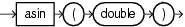Purpose

`asin` returns the arc sine of a `double` angle, in the range of `-pi/2` through `pi/2`, as a `double`.

For more information, see `http://java.sun.com/javase/6/docs/api/java/lang/Math.html#asin(double)`.

Examples

Consider the query `q74` in Example 12-16. Given the data stream `SFunc` with schema `(c1 integer, c2 double, c3 bigint)` in Example 12-17, the query returns the stream in Example 12-18.

Example 12-16 asin Function Query

```<query id="q74"><![CDATA[
select asin(c2) from SFunc
]]></query>
```

Example 12-17 asin Function Stream Input

```Timestamp   Tuple
10        1,0.5,8
1000        4,0.7,6
1200        3,0.89,12
2000        8,0.4,4
```

Example 12-18 asin Function Stream Output

```Timestamp   Tuple Kind  Tuple
10:       +           0.5235988
1000:       +           0.7753975
1200:       +           1.0973451
2000:       +           0.41151685
```

## atan

Syntax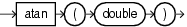Purpose

`atan` returns the arc tangent of a `double` angle, in the range of `-pi/2` through `pi/2,` as a `double`.

For more information, see `http://java.sun.com/javase/6/docs/api/java/lang/Math.html#atan(double)`.

Examples

Consider the query `q75` in Example 12-19. Given the data stream `SFunc` with schema `(c1 integer, c2 double, c3 bigint)` in Example 12-20, the query returns the stream in Example 12-21.

Example 12-19 atan Function Query

```<query id="q75"><![CDATA[
select atan(c2) from SFunc
]]></query>
```

Example 12-20 atan Function Stream Input

```Timestamp   Tuple
10        1,0.5,8
1000        4,0.7,6
1200        3,0.89,12
2000        8,0.4,4
```

Example 12-21 atan Function Stream Output

```Timestamp   Tuple Kind  Tuple
10:       +           0.4636476
1000:       +           0.61072594
1200:       +           0.7272627
2000:       +           0.3805064
```

## atan2

Syntax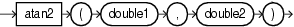Purpose

`atan2` converts rectangular coordinates (`x,y`) to polar (`r,theta`) coordinates.

This function takes the following arguments:

• `double1`: the ordinate coordinate.

• `double2`: the abscissa coordinate.

This function returns the theta component of the point (`r,theta`) in polar coordinates that corresponds to the point (`x,y`) in Cartesian coordinates as a `double`.

For more information, see `http://java.sun.com/javase/6/docs/api/java/lang/Math.html#atan2(double,%20double)`.

Examples

Consider the query `q63` in Example 12-22. Given the data stream `SFunc` with schema `(c1 integer, c2 double, c3 bigint)` in Example 12-23, the query returns the stream in Example 12-24.

Example 12-22 atan2 Function Query

```<query id="q63"><![CDATA[
select atan2(c2,c2) from SFunc
]]></query>
```

Example 12-23 atan2 Function Stream Input

```Timestamp   Tuple
10        1,0.5,8
1000        4,0.7,6
1200        3,0.89,12
2000        8,0.4,4
```

Example 12-24 atan2 Function Stream Output

```Timestamp   Tuple Kind  Tuple
10:       +           0.7853982
1000:       +           0.7853982
1200:       +           0.7853982
2000:       +           0.7853982
```

## cbrt

Syntax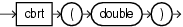Purpose

`cbrt` returns the cube root of the `double` argument as a `double`.

For positive finite `a`, `cbrt(-a) == -cbrt(a)`; that is, the cube root of a negative value is the negative of the cube root of that value's magnitude.

For more information, see `http://java.sun.com/javase/6/docs/api/java/lang/Math.html#cbrt(double)`.

Examples

Consider the query `q76` in Example 12-25. Given the data stream `SFunc` with schema `(c1 integer, c2 float, c3 bigint)` in Example 12-26, the query returns the stream in Example 12-27.

Example 12-25 cbrt Function Query

```<query id="q76"><![CDATA[
select cbrt(c2) from SFunc
]]></query>
```

Example 12-26 cbrt Function Stream Input

```Timestamp   Tuple
10        1,0.5,8
1000        4,0.7,6
1200        3,0.89,12
2000        8,0.4,4
```

Example 12-27 cbrt Function Stream Output

```Timestamp   Tuple Kind  Tuple
10:       +           0.7937005
1000:       +           0.887904
1200:       +           0.9619002
2000:       +           0.73680633
```

## ceil1

Syntax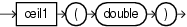Purpose

`ceil1` returns the smallest (closest to negative infinity) `double` value that is greater than or equal to the `double` argument and equals a mathematical integer.

To avoid possible rounding error, consider using `(long) cern.jet.math.Arithmetic.ceil(double)`.

• `http://java.sun.com/javase/6/docs/api/java/lang/Math.html#ceil(double)`

• "ceil"

Examples

Consider the query `q77` in Example 12-28. Given the data stream `SFunc` with schema `(c1 integer, c2 double, c3 bigint)` in Example 12-29, the query returns the stream in Example 12-30.

Example 12-28 ceil1 Function Query

```<query id="q77"><![CDATA[
select ceil1(c2) from SFunc
]]></query>
```

Example 12-29 ceil1 Function Stream Input

```Timestamp   Tuple
10        1,0.5,8
1000        4,0.7,6
1200        3,0.89,12
2000        8,0.4,4
```

Example 12-30 ceil1 Function Stream Output

```Timestamp   Tuple Kind  Tuple
10:       +           1.0
1000:       +           1.0
1200:       +           1.0
2000:       +           1.0
```

## cos

Syntax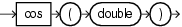Purpose

`cos` returns the trigonometric cosine of a `double` angle as a `double`.

For more information, see `http://java.sun.com/javase/6/docs/api/java/lang/Math.html#cos(double)`.

Examples

Consider the query `q61` in Example 12-31. Given the data stream `SFunc` with schema `(c1 integer, c2 double, c3 bigint)` in Example 12-32, the query returns the stream in Example 12-33.

Example 12-31 cos Function Query

```<query id="q61"><![CDATA[
select cos(c2) from SFunc
]]></query>
```

Example 12-32 cos Function Stream Input

```Timestamp   Tuple
10        1,0.5,8
1000        4,0.7,6
1200        3,0.89,12
2000        8,0.4,4
```

Example 12-33 cos Function Stream Output

```Timestamp   Tuple Kind  Tuple
10:       +           0.87758255
1000:       +           0.7648422
1200:       +           0.62941206
2000:       +           0.921061
```

## cosh

Syntax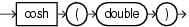Purpose

`cosh` returns the hyperbolic cosine of a `double` value as a `double`.

For more information, see `http://java.sun.com/javase/6/docs/api/java/lang/Math.html#cosh(double)`.

Examples

Consider the query `q78` in Example 12-34. Given the data stream `SFunc` with schema `(c1 integer, c2 double, c3 bigint)` in Example 12-35, the query returns the stream in Example 12-36.

Example 12-34 cosh Function Query

```<query id="q78"><![CDATA[
select cosh(c2) from SFunc
]]></query>
```

Example 12-35 cosh Function Stream Input

```Timestamp   Tuple
10        1,0.5,8
1000        4,0.7,6
1200        3,0.89,12
2000        8,0.4,4
```

Example 12-36 cosh Function Stream Output

```Timestamp   Tuple Kind  Tuple
10:       +           1.127626
1000:       +           1.255169
1200:       +           1.4228927
2000:       +           1.0810723
```

## exp

Syntax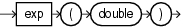Purpose

`exp` returns Euler's number e raised to the power of the `double` argument as a `double`.

Note that for values of `x` near 0, the exact sum of `expm1(x) + 1` is much closer to the true result of Euler's number e raised to the power of `x` than `EXP(x)`.

• `http://java.sun.com/javase/6/docs/api/java/lang/Math.html#exp(double)`

• "expm1"

Examples

Consider the query `q79` in Example 12-37. Given the data stream `SFunc` with schema `(c1 integer, c2 double, c3 bigint)` in Example 12-38, the query returns the stream in Example 12-39.

Example 12-37 exp Function Query

```<query id="q79"><![CDATA[
select exp(c2) from SFunc
]]></query>
```

Example 12-38 exp Function Stream Input

```Timestamp   Tuple
10        1,0.5,8
1000        4,0.7,6
1200        3,0.89,12
2000        8,0.4,4
```

Example 12-39 exp Function Stream Output

```Timestamp   Tuple Kind  Tuple
10:       +           1.6487212
1000:       +           2.0137527
1200:       +           2.4351296
2000:       +           1.4918247
```

## expm1

Syntax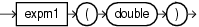Purpose

`expm1` returns the computation that Figure 12-1 shows as a `double`, where `x` is the `double` argument and e is Euler's number.

Figure 12-1 java.lang.Math Expm1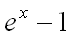Note that for values of `x` near 0, the exact sum of `expm1(x) + 1` is much closer to the true result of Euler's number e raised to the power of `x` than `exp(x)`.

• `http://java.sun.com/javase/6/docs/api/java/lang/Math.html#expm1(double)`

• "exp"

Examples

Consider the query `q80` in Example 12-40. Given the data stream `SFunc` with schema `(c1 integer, c2 double, c3 bigint)` in Example 12-41, the query returns the stream in Example 12-42.

Example 12-40 expm1 Function Query

```<query id="q80"><![CDATA[
select expm1(c2) from SFunc
]]></query>
```

Example 12-41 expm1 Function Stream Input

```Timestamp   Tuple
10        1,0.5,8
1000        4,0.7,6
1200        3,0.89,12
2000        8,0.4,4
```

Example 12-42 expm1 Function Stream Output

```Timestamp   Tuple Kind  Tuple
10:       +           0.6487213
1000:       +           1.0137527
1200:       +           1.4351296
2000:       +           0.49182472
```

## floor1

Syntax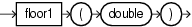Purpose

`floor1` returns the largest (closest to positive infinity) `double` value that is less than or equal to the `double` argument and equals a mathematical integer.

To avoid possible rounding error, consider using `(long) cern.jet.math.Arithmetic.floor(double)`.

• `http://java.sun.com/javase/6/docs/api/java/lang/Math.html#floor(double)`

• "floor"

Examples

Consider the query `q81` in Example 12-43. Given the data stream `SFunc` with schema `(c1 integer, c2 double, c3 bigint)` in Example 12-44, the query returns the stream in Example 12-45.

Example 12-43 floor1 Function Query

```<query id="q81"><![CDATA[
select floor1(c2) from SFunc
]]></query>
```

Example 12-44 floor1 Function Stream Input

```Timestamp   Tuple
10        1,0.5,8
1000        4,0.7,6
1200        3,0.89,12
2000        8,0.4,4
```

Example 12-45 floor1 Function Stream Output

```Timestamp   Tuple Kind  Tuple
10:       +           0.0
1000:       +           0.0
1200:       +           0.0
2000:       +           0.0
```

## hypot

Syntax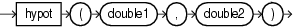Purpose

`hypot` returns the hypotenuse (see Figure 12-2) of the `double` arguments as a `double`.

Figure 12-2 java.lang.Math hypot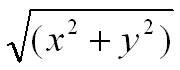This function takes the following arguments:

• `double1`: the `x` value.

• `double2`: the `y` value.

The hypotenuse is computed without intermediate overflow or underflow.

For more information, see `http://java.sun.com/javase/6/docs/api/java/lang/Math.html#hypot(double,%20double)`.

Examples

Consider the query `q82` in Example 12-46. Given the data stream `SFunc` with schema `(c1 integer, c2 double, c3 bigint)` in Example 12-47, the query returns the stream in Example 12-48.

Example 12-46 hypot Function Query

```<query id="q82"><![CDATA[
select hypot(c2,c2) from SFunc
]]></query>
```

Example 12-47 hypot Function Stream Input

```Timestamp   Tuple
10        1,0.5,8
1000        4,0.7,6
1200        3,0.89,12
2000        8,0.4,4
```

Example 12-48 hypot Function Stream Output

```Timestamp   Tuple Kind  Tuple
10:       +           0.70710677
1000:       +           0.98994946
1200:       +           1.2586501
2000:       +           0.56568545
```

## IEEEremainder

Syntax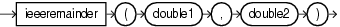Purpose

`IEEEremainder` computes the remainder operation on two `double` arguments as prescribed by the IEEE 754 standard and returns the result as a `double`.

This function takes the following arguments:

• `double1`: the dividend.

• `double2`: the divisor.

The remainder value is mathematically equal to `f1 - f2 × n`, where `n` is the mathematical integer closest to the exact mathematical value of the quotient `f1/f2`, and if two mathematical integers are equally close to `f1/f2`, then `n` is the integer that is even. If the remainder is zero, its sign is the same as the sign of the first argument.

For more information, see `http://java.sun.com/javase/6/docs/api/java/lang/Math.html#IEEEremainder(double,%20double)`.

Examples

Consider the query `q72` in Example 12-49. Given the data stream `SFunc` with schema `(c1 integer, c2 double, c3 bigint)` in Example 12-50, the query returns the stream in Example 12-51.

Example 12-49 IEEEremainder Function Query

```<query id="q72"><![CDATA[
select IEEEremainder(c2,c2) from SFunc
]]></query>
```

Example 12-50 IEEEremainder Function Stream Input

```Timestamp   Tuple
10        1,0.5,8
1000        4,0.7,6
1200        3,0.89,12
2000        8,0.4,4
```

Example 12-51 IEEEremainder Function Stream Output

```Timestamp   Tuple Kind  Tuple
10:       +           0.0
1000:       +           0.0
1200:       +           0.0
2000:       +           0.0
```

## log1

Syntax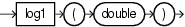Purpose

`log1` returns the natural logarithm (base e) of a `double` value as a `double`.

Note that for small values `x`, the result of `log1p(x)` is much closer to the true result of `ln(1 + x)` than the floating-point evaluation of `log(1.0+x)`.

• `http://java.sun.com/javase/6/docs/api/java/lang/Math.html#log(double)`

• "log1p"

Examples

Consider the query `q83` in Example 12-52. Given the data stream `SFunc` with schema `(c1 integer, c2 double, c3 bigint)` in Example 12-53, the query returns the stream in Example 12-54.

Example 12-52 log1 Function Query

```<query id="q83"><![CDATA[
select log1(c2) from SFunc
]]></query>
```

Example 12-53 log1 Function Stream Input

```Timestamp   Tuple
10        1,0.5,8
1000        4,0.7,6
1200        3,0.89,12
2000        8,0.4,4
```

Example 12-54 log1 Function Stream Output

```Timestamp   Tuple Kind  Tuple
10:       +           -0.6931472
1000:       +           -0.35667497
1200:       +           -0.11653383
2000:       +           -0.9162907
```

## log101

Syntax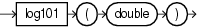Purpose

`log101` returns the base 10 logarithm of a `double` value as a `double`.

For more information, see `http://java.sun.com/javase/6/docs/api/java/lang/Math.html#log10(double)`.

Examples

Consider the query `q84` in Example 12-55. Given the data stream `SFunc` with schema `(c1 integer, c2 double, c3 bigint)` in Example 12-56, the query returns the stream in Example 12-57.

Example 12-55 log101 Function Query

```<query id="q84"><![CDATA[
select log101(c2) from SFunc
]]></query>
```

Example 12-56 log101 Function Stream Input

```Timestamp   Tuple
10        1,0.5,8
1000        4,0.7,6
1200        3,0.89,12
2000        8,0.4,4
```

Example 12-57 log101 Function Stream Output

```Timestamp   Tuple Kind  Tuple
10:       +           -0.30103
1000:       +           -0.15490197
1200:       +           -0.050610002
2000:       +           -0.39794
```

## log1p

Syntax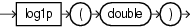Purpose

`log1p` returns the natural logarithm of the sum of the `double` argument and 1 as a `double`.

Note that for small values `x`, the result of `log1p(x)` is much closer to the true result of `ln(1 + x)` than the floating-point evaluation of `log(1.0+x)`.

• `http://java.sun.com/javase/6/docs/api/java/lang/Math.html#log1p(double)`

• "log1"

Examples

Consider the query `q85` in Example 12-58. Given the data stream `SFunc` with schema `(c1 integer, c2 double, c3 bigint)` in Example 12-59, the query returns the stream in Example 12-60.

Example 12-58 log1p Function Query

```<query id="q85"><![CDATA[
select log1p(c2) from SFunc
]]></query>
```

Example 12-59 log1p Function Stream Input

```Timestamp   Tuple
10        1,0.5,8
1000        4,0.7,6
1200        3,0.89,12
2000        8,0.4,4
```

Example 12-60 log1p Function Stream Output

```Timestamp   Tuple Kind  Tuple
10:       +           0.4054651
1000:       +           0.53062826
1200:       +           0.63657683
2000:       +           0.33647224
```

## pow

Syntax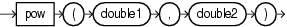Purpose

`pow` returns the value of the first `double` argument (the base) raised to the power of the second `double` argument (the exponent) as a `double`.

This function takes the following arguments:

• `double1`: the base.

• `double2`: the exponent.

For more information, see `http://java.sun.com/javase/6/docs/api/java/lang/Math.html#pow(double,%20double)`.

Examples

Consider the query `q65` in Example 12-61. Given the data stream `SFunc` with schema `(c1 integer, c2 double, c3 bigint)` in Example 12-62, the query returns the stream in Example 12-63.

Example 12-61 pow Function Query

```<query id="q65"><![CDATA[
select pow(c2,c2) from SFunc
]]></query>
```

Example 12-62 pow Function Stream Input

```Timestamp   Tuple
10        1,0.5,8
1000        4,0.7,6
1200        3,0.89,12
2000        8,0.4,4
```

Example 12-63 pow Function Stream Output

```Timestamp   Tuple Kind  Tuple
10:       +           0.70710677
1000:       +           0.7790559
1200:       +           0.9014821
2000:       +           0.69314486
```

## rint

Syntax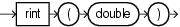Purpose

`rint` returns the `double` value that is closest in value to the `double` argument and equals a mathematical integer. If two `double` values that are mathematical integers are equally close, the result is the integer value that is even.

For more information, see `http://java.sun.com/javase/6/docs/api/java/lang/Math.html#rint(double)`.

Examples

Consider the query `q86` in Example 12-64. Given the data stream `SFunc` with schema `(c1 integer, c2 double, c3 bigint)` in Example 12-65, the query returns the stream in Example 12-66.

Example 12-64 rint Function Query

```<query id="q86"><![CDATA[
select rint(c2) from SFunc
]]></query>
```

Example 12-65 rint Function Stream Input

```Timestamp   Tuple
10        1,0.5,8
1000        4,0.7,6
1200        3,0.89,12
2000        8,0.4,4
```

Example 12-66 rint Function Stream Output

```Timestamp   Tuple Kind  Tuple
10:       +           0.0
1000:       +           1.0
1200:       +           1.0
2000:       +           0.0
```

## round

Syntax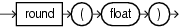Purpose

`round` returns the closest `integer` to the `float` argument.

For more information, see `http://java.sun.com/javase/6/docs/api/java/lang/Math.html#round(float)`.

Examples

Consider the query `q87` in Example 12-67. Given the data stream `SFunc` with schema `(c1 integer, c2 double, c3 bigint)` in Example 12-68, the query returns the stream in Example 12-69.

Example 12-67 round Function Query

```<query id="q87"><![CDATA[
select round(c2) from SFunc
]]></query>
```

Example 12-68 round Function Stream Input

```Timestamp   Tuple
10        1,0.5,8
1000        4,0.7,6
1200        3,0.89,12
2000        8,0.4,4
```

Example 12-69 round Function Stream Output

```Timestamp   Tuple Kind  Tuple
10:       +           1
1000:       +           1
1200:       +           1
2000:       +           0
```

## round1

Syntax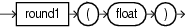Purpose

`round1` returns the closest `integer` to the `float` argument.

For more information, see `http://java.sun.com/javase/6/docs/api/java/lang/Math.html#round(float)`.

Examples

Consider the query `q88` in Example 12-70. Given the data stream `SFunc` with schema `(c1 integer, c2 double, c3 bigint)` in Example 12-71, the query returns the stream in Example 12-72.

Example 12-70 round1 Function Query

```<query id="q88"><![CDATA[
select round1(c2) from SFunc
]]></query>
```

Example 12-71 round1 Function Stream Input

```Timestamp   Tuple
10        1,0.5,8
1000        4,0.7,6
1200        3,0.89,12
2000        8,0.4,4
```

Example 12-72 round1 Function Stream Output

```Timestamp   Tuple Kind  Tuple
10:       +           1
1000:       +           1
1200:       +           1
2000:       +           0
```

## signum

Syntax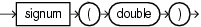Purpose

`signum` returns the signum function of the `double` argument as a `double`:

• zero if the argument is zero

• 1.0 if the argument is greater than zero

• -1.0 if the argument is less than zero

For more information, see `http://java.sun.com/javase/6/docs/api/java/lang/Math.html#signum(double)`.

Examples

Consider the query `q70` in Example 12-73. Given the data stream `SFunc` with schema `(c1 integer, c2 double, c3 bigint)` in Example 12-74, the query returns the stream in Example 12-75.

Example 12-73 signum Function Query

```<query id="q70"><![CDATA[
select signum(c2) from SFunc
]]></query>
```

Example 12-74 signum Function Stream Input

```Timestamp   Tuple
10        1,0.5,8
1000        4,-0.7,6
1200        3,-0.89,12
2000        8,0.4,4
```

Example 12-75 signum Function Stream Output

```Timestamp   Tuple Kind  Tuple
10:       +           1.0
1000:       +           -1.0
1200:       +           -1.0
2000:       +           1.0
```

## signum1

Syntax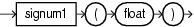Purpose

`signum1` returns the signum function of the `float` argument as a `float`:

• zero if the argument is zero

• 1.0 if the argument is greater than zero

• -1.0 if the argument is less than zero

For more information, see `http://java.sun.com/javase/6/docs/api/java/lang/Math.html#signum(float)`.

Examples

Consider the query `q71` in Example 12-76. Given the data stream `SFunc` with schema `(c1 integer, c2 double, c3 bigint)` in Example 12-77, the query returns the relation in Example 12-78.

Example 12-76 signum1 Function Query

```<query id="q71"><![CDATA[
select signum1(c2) from SFunc
]]></query>
```

Example 12-77 signum1 Function Stream Input

```Timestamp   Tuple
10        1,0.5,8
1000        4,-0.7,6
1200        3,-0.89,12
2000        8,0.4,4
```

Example 12-78 signum1 Function Relation Output

```Timestamp   Tuple Kind  Tuple
10:       +           1.0
1000:       +           -1.0
1200:       +           -1.0
2000:       +           1.0
```

## sin

Syntax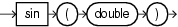Purpose

`sin` returns the trigonometric sine of a `double` angle as a `double`.

For more information, see `http://java.sun.com/javase/6/docs/api/java/lang/Math.html#sin(double)`.

Examples

Consider the query `q60` in Example 12-79. Given the data stream `SFunc` with schema (`c1 integer`, `c2 float`, `c3 bigint`) in Example 12-80, the query returns the stream in Example 12-81.

Example 12-79 sin Function Query

```<query id="q60"><![CDATA[
select sin(c2) from SFunc
]]></query>
```

Example 12-80 sin Function Stream Input

```Timestamp   Tuple
10        1,0.5,8
1000        4,0.7,6
1200        3,0.89,12
2000        8,0.4,4
```

Example 12-81 sin Function Stream Output

```Timestamp   Tuple Kind  Tuple
10:       +           0.47942555
1000:       +           0.64421767
1200:       +           0.7770717
2000:       +           0.38941833
```

## sinh

Syntax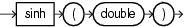Purpose

`sinh` returns the hyperbolic sine of a `double` value as a `double`.

For more information, see `http://java.sun.com/javase/6/docs/api/java/lang/Math.html#sinh(double)`.

Examples

Consider the query `q89` in Example 12-82. Given the data stream `SFunc` with schema `(c1 integer, c2 double, c3 bigint)` in Example 12-83, the query returns the stream in Example 12-84.

Example 12-82 sinh Function Query

```<query id="q89"><![CDATA[
select sinh(c2) from SFunc
]]></query>
```

Example 12-83 sinh Function Stream Input

```Timestamp   Tuple
10        1,0.5,8
1000        4,0.7,6
1200        3,0.89,12
2000        8,0.4,4
```

Example 12-84 sinh Function Stream Output

```Timestamp   Tuple Kind  Tuple
10:       +           0.5210953
1000:       +           0.75858366
1200:       +           1.012237
2000:       +           0.41075233
```

## sqrt

Syntax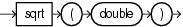Purpose

`sqrt` returns the correctly rounded positive square root of a `double` value as a `double`.

For more information, see `http://java.sun.com/javase/6/docs/api/java/lang/Math.html#sqrt(double)`.

Examples

Consider the query `q64` in Example 12-85. Given the data stream `SFunc` with schema (`c1 integer`, `c2 float`, `c3 bigint`) in Example 12-86, the query returns the stream in Example 12-87.

Example 12-85 sqrt Function Query

```<query id="q64"><![CDATA[
select sqrt(c2) from SFunc
]]></query>
```

Example 12-86 sqrt Function Stream Input

```Timestamp   Tuple
10        1,0.5,8
1000        4,0.7,6
1200        3,0.89,12
2000        8,0.4,4
```

Example 12-87 sqrt Function Stream Output

```Timestamp   Tuple Kind  Tuple
10:       +           0.70710677
1000:       +           0.83666
1200:       +           0.9433981
2000:       +           0.6324555
```

## tan

Syntax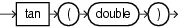Purpose

`tan` returns the trigonometric tangent of a `double` angle as a `double`.

For more information, see `http://java.sun.com/javase/6/docs/api/java/lang/Math.html#tan(double)`.

Examples

Consider the query `q62` in Example 12-88. Given the data stream `SFunc` with schema `(c1 integer, c2 double, c3 bigint)` in Example 12-89, the query returns the stream in Example 12-90.

Example 12-88 tan Function Query

```<query id="q62"><![CDATA[
select tan(c2) from SFunc
]]></query>
```

Example 12-89 tan Function Stream Input

```Timestamp   Tuple
10        1,0.5,8
1000        4,0.7,6
1200        3,0.89,12
2000        8,0.4,4
```

Example 12-90 tan Function Stream Output

```Timestamp   Tuple Kind  Tuple
10:       +           0.5463025
1000:       +           0.8422884
1200:       +           1.2345995
2000:       +           0.42279324
```

## tanh

Syntax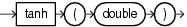Purpose

`tanh` returns the hyperbolic tangent of a `double` value as a `double`.

For more information, see `http://java.sun.com/javase/6/docs/api/java/lang/Math.html#tanh(double)`.

Examples

Consider the query `q90` in Example 12-91. Given the data stream `SFunc` with schema `(c1 integer, c2 double, c3 bigint)` in Example 12-92, the query returns the stream in Example 12-93.

Example 12-91 tanh Function Query

```<query id="q90"><![CDATA[
select tanh(c2) from SFunc
]]></query>
```

Example 12-92 tanh Function Stream Input

```Timestamp   Tuple
10        1,0.5,8
1000        4,0.7,6
1200        3,0.89,12
2000        8,0.4,4
```

Example 12-93 tanh Function Stream Output

```Timestamp   Tuple Kind  Tuple
10:       +           0.46211717
1000:       +           0.6043678
1200:       +           0.7113937
2000:       +           0.37994897
```

## todegrees

Syntax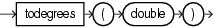Purpose

`todegrees` converts a `double` angle measured in radians to an approximately equivalent angle measured in degrees as a `double`.

The conversion from radians to degrees is generally inexact; do not expect `COS(TORADIANS(90.0))` to exactly equal `0.0`.

• `http://java.sun.com/javase/6/docs/api/java/lang/Math.html#toDegrees(double)`

• "cos"

Examples

Consider the query `q91` in Example 12-94. Given the data stream `SFunc` with schema `(c1 integer, c2 double, c3 bigint)` in Example 12-95, the query returns the stream in Example 12-96.

Example 12-94 todegrees Function Query

```<query id="q91"><![CDATA[
select todegrees(c2) from SFunc
]]></query>
```

Example 12-95 todegrees Function Stream Input

```Timestamp   Tuple
10        1,0.5,8
1000        4,0.7,6
1200        3,0.89,12
2000        8,0.4,4
```

Example 12-96 todegrees Function Stream Output

```Timestamp   Tuple Kind  Tuple
10:       +           28.64789
1000:       +           40.107044
1200:       +           50.993244
2000:       +           22.918312
```

Syntax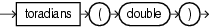Purpose

`toradians` converts a `double` angle measured in degrees to an approximately equivalent angle measured in radians as a `double`.

• `http://java.sun.com/javase/6/docs/api/java/lang/Math.html#toRadians(double)`

• "todegrees"

• "cos"

Examples

Consider the query `q92` in Example 12-97. Given the data stream `SFunc` with schema `(c1 integer, c2 double, c3 bigint)` in Example 12-98, the query returns the stream in Example 12-99.

```<query id="q92"><![CDATA[
]]></query>
```

Example 12-98 toradians Function Stream Input

```Timestamp   Tuple
10        1,0.5,8
1000        4,0.7,6
1200        3,0.89,12
2000        8,0.4,4
```

Example 12-99 toradians Function Stream Output

```Timestamp   Tuple Kind  Tuple
10:       +           0.008726646
1000:       +           0.012217305
1200:       +           0.0155334305
2000:       +           0.006981317
```

## ulp

Syntax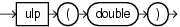Purpose

`ulp` returns the size of an ulp of the `double` argument as a `double`. In this case, an ulp of the argument value is the positive distance between this floating-point value and the double value next larger in magnitude.

For more information, see `http://java.sun.com/javase/6/docs/api/java/lang/Math.html#ulp(double)`.

Examples

Consider the query `q93` in Example 12-100. Given the data stream `SFunc` with schema `(c1 integer, c2 double, c3 bigint)` in Example 12-101, the query returns the stream in Example 12-102.

Example 12-100 ulp Function Query

```<query id="q93"><![CDATA[
select ulp(c2) from SFunc
]]></query>
```

Example 12-101 ulp Function Stream Input

```Timestamp   Tuple
10        1,0.5,8
1000        4,0.7,6
1200        3,0.89,12
2000        8,0.4,4
```

Example 12-102 ulp Function Stream Output

```Timestamp   Tuple Kind  Tuple
10:       +           1.110223E-16
1000:       +           1.110223E-16
1200:       +           1.110223E-16
2000:       +           5.551115E-17
```

## ulp1

Syntax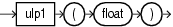Purpose

`ulp1` returns the size of an ulp of the `float` argument as a `float`. An ulp of a float value is the positive distance between this floating-point value and the float value next larger in magnitude.

For more information, see `http://java.sun.com/javase/6/docs/api/java/lang/Math.html#ulp(float)`.

Examples

Consider the query `q94` in Example 12-103. Given the data stream `SFunc` with schema `(c1 integer, c2 double, c3 bigint)` in Example 12-104, the query returns the relation in Example 12-105.

Example 12-103 ulp1 Function Query

```<query id="q94"><![CDATA[
select ulp1(c2) from SFunc
]]></query>
```

Example 12-104 ulp1 Function Stream Input

```Timestamp   Tuple
10        1,0.5,8
1000        4,0.7,6
1200        3,0.89,12
2000        8,0.4,4
```

Example 12-105 ulp1 Function Relation Output

```Timestamp   Tuple Kind  Tuple
10:       +           5.9604645E-8
1000:       +           5.9604645E-8
1200:       +           5.9604645E-8
2000:       +           2.9802322E-8
```